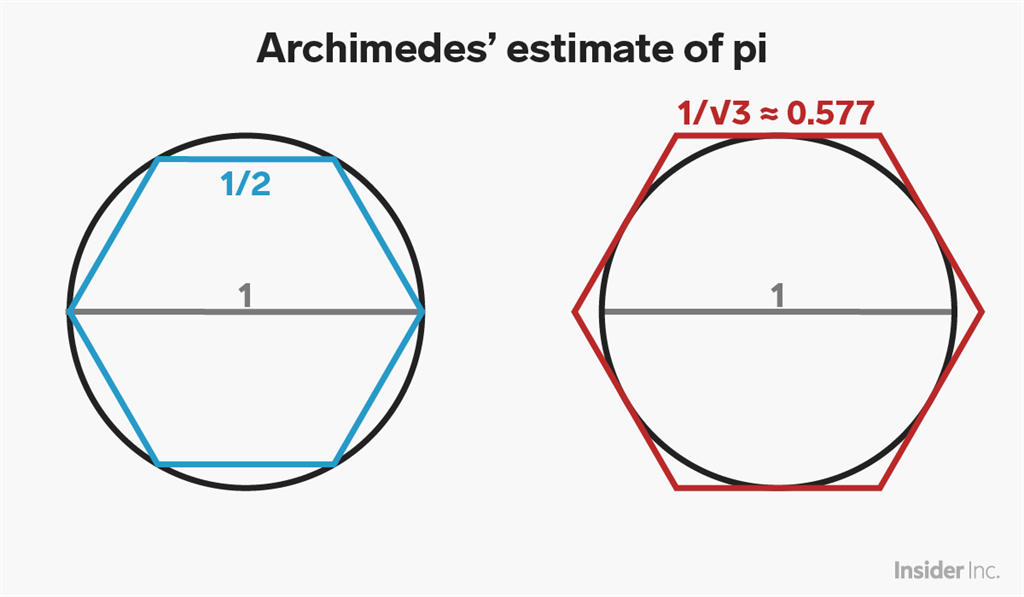March 14 is Pi Day.

• March 14 is Pi Day in the US, as the date matches the first three digits of the famous number.
• On Pi Day 2015, Google announced that a researcher had uncovered the first 31 trillion digits of pi, using a cloud-based computer algorithm.
• Ancient mathematicians like the Greek Archimedes used clever geometry tricks to come up with earlier estimates of p.

March 14 is Pi day, or in American notation, 3/14, matching the first three digits of p.

One of the fundamental constants of mathematics, p, is the ratio of a circle's circumference to its diameter.

It's an example of an irrational number - p can never be written as a fraction of two whole numbers, and it does not have a terminating or repeating decimal expansion. The decimal expansion of p goes on forever, never showing any repeating pattern. Since p is irrational, all we can ever hope to do is get better and better decimal approximations.

Indeed, on Pi Day 2019, Google researchers announced that they had found the first 31 trillion or so digits of p, setting a new record.

So, how did the ancients, who did not have access to cloud-based supercomputers like the Google engineers, first approximate p?

### How they found p

The Greek mathematician Archimedes developed one of the first somewhat-rigorous approaches to approximating p. Archimedes observed that polygons drawn inside and outside a circle would have perimeters somewhat close to the circumference of the circle.

As described in Jorg Arndt and Cristoph Haenel's book Pi Unleashed, Archimedes started with hexagons:Archimedes started with hexagons.

We start with a circle of diameter equal to one, so that, by definition, its circumference will equal p. Using some basic geometry and trigonometry, Archimedes observed that the length of each of the sides of the inscribed blue hexagon would be 1/2, and the lengths of the sides of the circumscribed red hexagon would be 1/v3.

The perimeter of the inscribed blue hexagon has to be smaller than the circumference of the circle, since the hexagon fits entirely inside the circle. The six sides of the hexagon all have length 1/2, so this perimeter is 6 × 1/2 = 3.

Similarly, the circumference of the circle has to be less than the perimeter of the circumscribed red hexagon, and this perimeter is 6 × 1/v3, which is about 3.46.

This gives us the inequalities 3 < p < 3.46, already moving us closer to 3.14.

Archimedes, through some further clever geometry, figured out how to estimate the perimeters for polygons with twice as many sides. He went from a 6-sided polygon, to a 12-sided polygon, to a 24-sided polygon, to a 48-sided polygon, and ended up with a 96-sided polygon. This final estimate gave a range for p between 3.1408 and 3.1428, which is accurate to two places.

Archimedes' method of approximating p with polygons, and similar techniques developed in China and India, would be the dominant way mathematicians would approach the calculation of the digits p for centuries.

Today, mathematicians like the Google researchers use algorithms based on the idea of infinite series from calculus, and our ever-faster computers allow us to find trillions of digits of p.